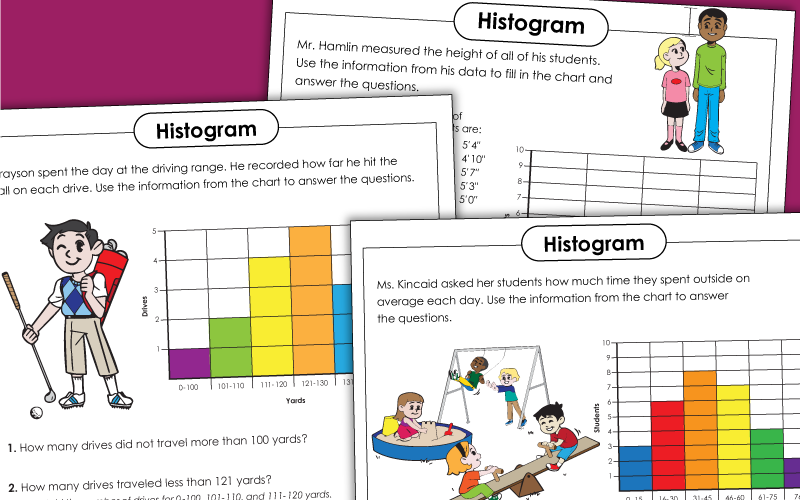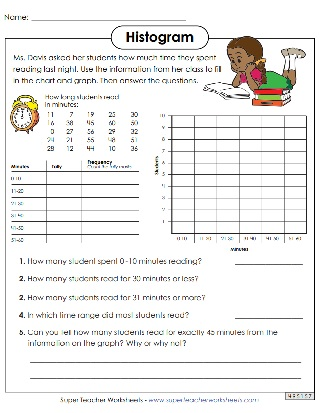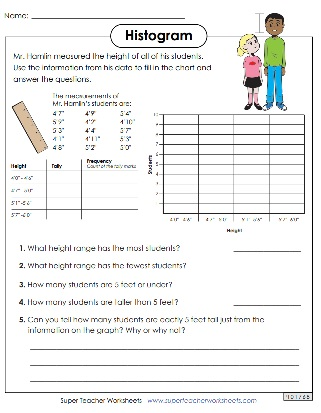# Histograms

Our collection of histogram worksheets helps students learn how to read and create this type of graph. Using given data, students can fill in histograms on their own and answer questions interpreting them.Students can use the data to fill in the histogram and then answer the questions about it to ensure they understand the information.
Students will create a histogram based on data provided about how long students read one night. Then they will answer questions about the graph and data.
With this worksheet, students can make a histogram using data provided about a track meet. Then they can answer questions regarding their graph and the data.
This histogram has information on a person's results from golfing at the driving range. Test your students' ability to understand histograms by having them complete this worksheet.
Use this worksheet to help students practice interpreting the information presented in a histogram.
With this worksheet, students will use the provided histogram to answer questions about how long a student practiced her violin.
Bar Graph Worksheets

Learn to read and interpret data on bar graphs with these printable PDFs.

More Graphing Worksheets

Practice reading and creating bar graphs, line graphs, line plots, pictographs, and pie graphs.

## Sample Worksheet ImagesMy Account
Site Information